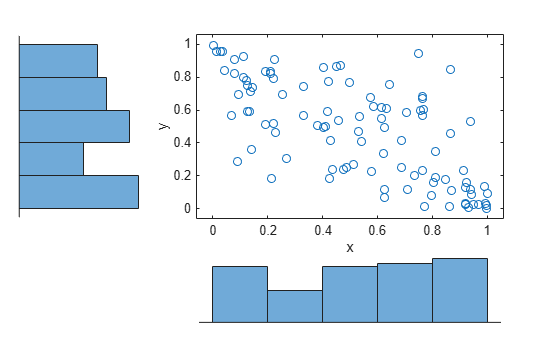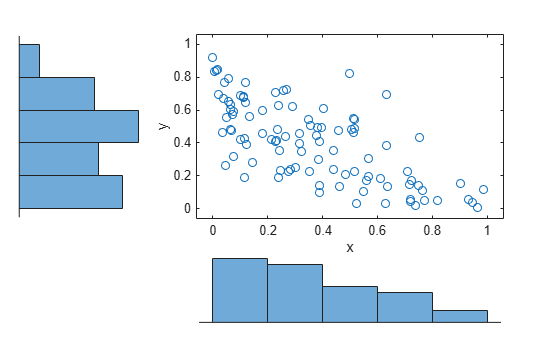# copularnd

Copula random numbers

## Syntax

``u = copularnd('Gaussian',rho,n)``
``u = copularnd('t',rho,nu,n)``
``u = copularnd(family,alpha,n)``

## Description

example

````u = copularnd('Gaussian',rho,n)` returns `n` random vectors generated from a Gaussian copula with linear correlation parameters `rho`.```
````u = copularnd('t',rho,nu,n)` returns `n` random vectors generated from a t copula with linear correlation parameters `rho` and degrees of freedom `nu`.```
````u = copularnd(family,alpha,n)` returns `n` random vectors generated from a bivariate Archimedean copula that has the type specified by `family` and the scalar parameter `alpha`.```

## Examples

collapse all

Generate correlated random data from a beta distribution using a bivariate Gaussian copula with Kendall's tau rank correlation equal to -0.5.

Compute the linear correlation parameter from the rank correlation value.

```rng default % For reproducibility tau = -0.5; rho = copulaparam('Gaussian',tau)```
```rho = -0.7071 ```

Use a Gaussian copula to generate a two-column matrix of dependent random values.

`u = copularnd('gaussian',rho,100);`

Each column contains 100 random values between 0 and 1, inclusive, sampled from a continuous uniform distribution.

Create a `scatterhist` plot to visualize the random numbers generated using the copula.

```figure scatterhist(u(:,1),u(:,2))```The histograms show that the data in each column of the copula has a marginal uniform distribution. The scatterplot shows that the data in the two columns is negatively correlated.

Use the inverse cdf function `betainv` to transform each column of the uniform marginal distributions into random numbers from a beta distribution. In the first column, the first shape parameter A is equal to 1, and a second shape parameter B is equal to 2. In the second column, the first shape parameter A is equal to 1.5, and a second shape parameter B is equal to 2.

`b = [betainv(u(:,1),1,2), betainv(u(:,2),1.5,2)];`

Create a `scatterhist` plot to visualize the correlated beta distribution data.

```figure scatterhist(b(:,1),b(:,2))```The histograms show the marginal beta distributions for each variable. The scatterplot shows the negative correlation.

Verify that the sample has a rank correlation approximately equal to the initial value for Kendall's tau.

`tau_sample = corr(b,'type','kendall')`
```tau_sample = 2×2 1.0000 -0.5135 -0.5135 1.0000 ```

The sample rank correlation of -0.5135 is approximately equal to the -0.5 initial value for tau.

## Input Arguments

collapse all

Linear correlation parameters for the copula, specified as a scalar value or matrix of scalar values.

• If `rho` is a p-by-p correlation matrix, then the output argument `u` is an n-by-p matrix.

• If `rho` is a scalar correlation coefficient, then the output argument `u` is an n-by-2 matrix.

Data Types: `single` | `double`

Number of random vectors to return, specified as a positive scalar value.

• If you specify the copula type as `'Gaussian'` or `'t'`, and `rho` is a p-by-p correlation matrix, then `u` is an n-by-p matrix.

• If you specify the copula type as `'Gaussian'` or `'t'`, and `rho` is a scalar correlation coefficient, then `u` is an n-by-2 matrix.

• If you specify the copula type as `'Clayton'`, `'Frank'`, or `'Gumbel'`, then `u` is an n-by-2 matrix.

Data Types: `single` | `double`

Degrees of freedom for the t copula, specified as a positive integer value.

Data Types: `single` | `double`

Bivariate Archimedean copula family, specified as one of the following.

 `'Clayton'` Clayton copula `'Frank'` Frank copula `'Gumbel'` Gumbel copula

Bivariate Archimedean copula parameter, specified as a scalar value. Permitted values for `alpha` depend on the specified copula family.

Copula FamilyPermitted Alpha Values
`'Clayton'`[0,∞)
`'Frank'`(-∞,∞)
`'Gumbel'`[1,∞)

Data Types: `single` | `double`

## Output Arguments

collapse all

Copula random numbers, returned as a matrix of scalar values. Each column of `u` is a sample from a `Uniform(0,1)` marginal distribution.

• If you specify the copula type as `'Gaussian'` or `'t'`, and `rho` is a p-by-p correlation matrix, then `u` is an n-by-p matrix.

• If you specify the copula type as `'Gaussian'` or `'t'`, and `rho` is a scalar correlation coefficient, then `u` is an n-by-2 matrix.

• If you specify the copula type as `'Clayton'`, `'Frank'`, or `'Gumbel'`, then `u` is an n-by-2 matrix.